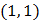# The incentre of the triangle formed by linesand, is at a)b)c)d)## Question ID - 50784 :- The incentre of the triangle formed by linesand, is at a)b)c)d)3537

(b)

Given equation of lines areandIncentre is on the line(Angled bisector ofand)Angle bisector ofandisandHere,internal bisector

So, intersection point ofandisThe required point of the incentre of triangle isNext Question :

A student obtained the following marks in percentages in his semester examination English 50, Maths 65, Statistics 70, Economics 58 and Accountancy 63. The weights of these subjects are 2, 2, 1, 1 and 1 respectively. What is the weighted arithmetic mean?

(a) 60                (b) 61             (c)  62           (d) 63